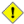### Using boost::units

odeint also works well with Boost.Units - a library for compile time unit and dimension analysis. It works by decoding unit information into the types of values. For a one-dimensional unit you can just use the Boost.Unit types as state type, deriv type and time type and hand the `vector_space_algebra` to the stepper definition and everything works just fine:

```typedef units::quantity< si::time , double > time_type;
typedef units::quantity< si::length , double > length_type;
typedef units::quantity< si::velocity , double > velocity_type;

typedef runge_kutta4< length_type , double , velocity_type , time_type ,
vector_space_algebra > stepper_type;
```

If you want to solve more-dimensional problems the individual entries typically have different units. That means that the `state_type` is now possibly heterogeneous, meaning that every entry might have a different type. To solve this problem, compile-time sequences from Boost.Fusion can be used.

To illustrate how odeint works with Boost.Units we use the harmonic oscillator as primary example. We start with defining all quantities

```#include <boost/numeric/odeint.hpp>
#include <boost/numeric/odeint/algebra/fusion_algebra.hpp>
#include <boost/numeric/odeint/algebra/fusion_algebra_dispatcher.hpp>

#include <boost/units/systems/si/length.hpp>
#include <boost/units/systems/si/time.hpp>
#include <boost/units/systems/si/velocity.hpp>
#include <boost/units/systems/si/acceleration.hpp>
#include <boost/units/systems/si/io.hpp>

#include <boost/fusion/container.hpp>

using namespace std;
using namespace boost::numeric::odeint;
namespace fusion = boost::fusion;
namespace units = boost::units;
namespace si = boost::units::si;

typedef units::quantity< si::time , double > time_type;
typedef units::quantity< si::length , double > length_type;
typedef units::quantity< si::velocity , double > velocity_type;
typedef units::quantity< si::acceleration , double > acceleration_type;
typedef units::quantity< si::frequency , double > frequency_type;

typedef fusion::vector< length_type , velocity_type > state_type;
typedef fusion::vector< velocity_type , acceleration_type > deriv_type;
```

Note, that the `state_type` and the `deriv_type` are now a compile-time fusion sequences. `deriv_type` represents x' and is now different from the state type as it has different unit definitions. Next, we define the ordinary differential equation which is completely equivalent to the example in Harmonic Oscillator:

```struct oscillator
{
frequency_type m_omega;

oscillator( const frequency_type &omega = 1.0 * si::hertz ) : m_omega( omega ) { }

void operator()( const state_type &x , deriv_type &dxdt , time_type t ) const
{
fusion::at_c< 0 >( dxdt ) = fusion::at_c< 1 >( x );
fusion::at_c< 1 >( dxdt ) = - m_omega * m_omega * fusion::at_c< 0 >( x );
}
};
```

Next, we instantiate an appropriate stepper. We must explicitly parametrize the stepper with the `state_type`, `deriv_type`, `time_type`.

```typedef runge_kutta_dopri5< state_type , double , deriv_type , time_type > stepper_type;

state_type x( 1.0 * si::meter , 0.0 * si::meter_per_second );

integrate_const( make_dense_output( 1.0e-6 , 1.0e-6 , stepper_type() ) , oscillator( 2.0 * si::hertz ) ,
x , 0.0 * si::second , 100.0 * si::second , 0.1 * si::second , streaming_observer( cout ) );
```

NoteWhen using compile-time sequences, the iteration over vector elements is done by the `fusion_algebra`, which is automatically chosen by odeint. For more on the state types / algebras see chapter Adapt your own state types.

It is quite easy but the compilation time might take very long. Furthermore, the observer is defined a bit different

```struct streaming_observer
{
std::ostream& m_out;

streaming_observer( std::ostream &out ) : m_out( out ) { }

struct write_element
{
std::ostream &m_out;
write_element( std::ostream &out ) : m_out( out ) { };

template< class T >
void operator()( const T &t ) const
{
m_out << "\t" << t;
}
};

template< class State , class Time >
void operator()( const State &x , const Time &t ) const
{
m_out << t;
fusion::for_each( x , write_element( m_out ) );
m_out << "\n";
}
};
```

CautionUsing Boost.Units works nicely but compilation can be very time and memory consuming. For example the unit test for the usage of Boost.Units in odeint take up to 4 GB of memory at compilation.

The full cpp file for this example can be found here harmonic_oscillator_units.cpp.